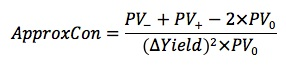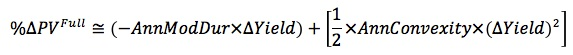### Why should I choose AnalystNotes?

AnalystNotes specializes in helping candidates pass. Period.

##### Subject 6. Bond Convexity
Duration is a first approximation of a bond's price or a portfolio's value to rate changes. It does a good job of estimating the percentage price change for a small change in interest rates but the estimation becomes poorer the larger the change in interest rates.

Duration always gives a lower than actual price, the reason being convexity. Thus, a convexity adjustment would take into account the curvature of the price/yield relationship in order to give a more accurate estimated price.

To improve the estimate provided by duration, particularly for a large change in yield, a convexity measure can be used.For a hypothetical 9%, 20-year bond selling to yield 6%, for a 20 basis point change in yield, P0 = 134.6722, P- = 137.5888, and P+ = 131.8439 ==> convexity measure = (131.8439 + 137.5888 - 2 x 134.6722)/(134.6722 x 0.0022) = 163.92.

Convexity indicates that as yield increases, the price of a bond declines at a declining rate. Given the convexity measure, the convexity adjustment to the duration estimate can be computed; the convexity adjustment is the amount that should be added to the duration estimate for the percentage price change.Consider a situation where you are using duration to compute the effect of a 250 basis point change in yield, where duration is 6.655 and the convexity adjustment is 1.8271. Using an estimate of modified duration, you determine that the percentage change in price of this bond resulting from a 250 basis point increase in yield should be 2.5 x -6.655 = -16.6375%. Adding the convexity adjustment, the percentage price should change by -16.6375 + 1.8271 = -14.8104%. Summarizing the price change estimates in response to the 250 basis point increase in yield, you have:

Duration estimate = -16.63750%
Total: - 14.8104%
The actual decrease is 14.95%, so the convexity adjustment does improve the estimate.

If you estimate the change resulting from a 250 basis point decrease in yield, the results can be summarized as:
Duration estimate: 16.6375%
Total: +18.4646%

The actual percentage increase in price is 18.62%. The convexity adjustment brings the modified duration estimate closer to the actual percentage change.

User Comment
Rotigga Convexity adjustment = (Convexity measure)*(change in yield)^2*100
cardinal08 As yield increases, price of a bond declines at a declining rate--> 2nd derivative
johntan1979 My finance professor taught me well NOT to round my intermediate steps.

In the example above, for good students (like what my professor would say it), the answer is 82.0621

BIG difference there, especially if we are talking about getting a more accurate estimation (isn't that the whole point about second approximation?)
johntan1979 This is where the STO and RCL functions come in very handy. To store a number, just press STO followed by any number you like from 0 to 9. To recall the number saved, just press RCL followed by the number you chose earlier.
Bill convexity calculation without 2 x in the denominator? mistake in the textbook?
rainerh I'm a litte confused about the definition for the Convexity Effect/Adjustment when calculating the price change when interest rates change.

The approximation of the Bond Value is nothing more than a second order Taylor Approximation and therefore the adjustment should be: -Duration x delta_y+1/2*convexity*delta_y^2

But sometimes the adjustment is calculated with -Duration x delta_y+convexity*delta_y^2.What about the 1/2?
haney In CFAI curriculum, the adjustment is�:��- Duration x delta_y + 1/2 convexity*delta_y^2

The 1/2 is necessary, as you say.� What CFA Institute doesn't tell you at Level I is that it's included in the convexity coefficient.

At Level II you'll learn that the calculation of (effective) convexity is: Ceff = [(P-) + (P+) - 2 � (P0)] / (2 � P0 � Δy)

Do not bother with this formula now; just know how to use the convexity term in the (percentage) price change calculation.
cbracho54 thanks haney!!!